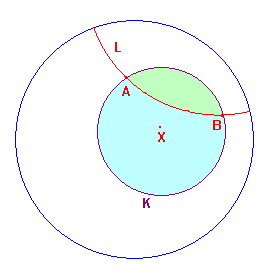# inscribed hyperbolic polygons

 Suppose that A and B lie on a hyperbolic circle K. The hyperbolic line AB must contain points outside K, so the hyperbolic segment AB cannot meet K between A and B. It follows that the line AB divides the circle K into two arcs, and the interior of K into two regions. If we suppose, further, that AB does not pass through X, the hyperbolic centre of K, then the region containing X is the major region, and its boundary the major arc. Note that these concepts are invariant under the hyperbolic group. To see this, note that P is in the minor region (arc) if and only if PX crosses AB. In euclidean geometry, we are familiar with results on angles lying on the same arc, or within the same region. Naturally, we seek hyperbolic analogues. Note: the euclidean and hyperbolic segments AB give the same arcs since they are defined by the points A and B. Since we are largely concerned with hyperbolic length and angle, we will introduce separate notation for the euclidean quantities : |AB| = euclidean distance between the points A and B. e(ABC) = angle at B in the euclidean triangle ABC.the hybrid angle theorem Suppose that A,B,C are points in the disk, with A ≠ B. If A,B,C lie on a circle K with centre O, then cos(e(ACB)) = (s2(AC)+s2(BC)-s2(AB))/2s(AC)s(BC). proof The euclidean lengths are given by the distances theorem. Now apply the euclidean cosine rule to ΔABC. The factors involving c(OA), c(OB), c(OC) cancel since OA,OB,OC are radii in both the euclidean and hyperbolic sense. Remark Since hyperbolic length of XY, and hence s(XY) are hyperbolic invariants, the quantity which appears on the right is also an invariant of the hyperbolic triangle ΔABC, though it will not correspond to the euclidean angle at C in general. Definition If A,B,C are distinct points on the disk, define E(A,B,C) = (s2(AC)+s2(BC)-s2(AB))/2s(AC)s(BC). The function E gives yet another characterization of circumcircles. More importantly, it aso allows us to describe the major and minor arcs when AB is not a hyperbolic diameter. Suppose that we apply a hyperbolic transformation so that the hyperbolic (and hence the euclidean) centre is at O. Then the major and minor arcs agree in both geometries. From the euclidean picture, C lies on ther major arc if and only if 0. We will also require the following algebraic result : the F-H relationIf F(x,y,z) = x2+y2-z2/2xy, then 1-F2(x,y,z) = H(x,y,z)/4x2y2. The proof is simply an algebraic manipulation from the given formulae for E and H. Note Since the (hyperbolic) centre is O, K is a also euclidean circle with centre O. We shall begin by looking at the case where AB is a diameter. the hyperbolic semi-circle theorem Suppose that A,B,C are points in the disk, with A ≠ B, and that K is the hyperbolic circle on AB as diameter. Then C lies on K if and only if s2(AC)+s2(BC) = s2(AB), inside K if and only if s2(AC)+s2(BC) < s2(AB), outside K if and only if s2(AC)+s2(BC) > s2(AB). proof We apply the origin lemma so the centre of the circle is O. Then AB is a euclidean diameter and so C lies on the circle if and only if e(ACB) = π/2, i.e. cos(e(ACB)) = 0, etc. (part (7)). The results now follow from the hybrid angle theorem. We can, of course also state the result in hybrid form: the hybrid semi-circle theorem Suppose that A,B,C are points in the disk, with A ≠ B. Then C lies on the hyperbolic circle with diameter AB if and only if the euclidean (s(AB),s(BC),s(CA)) triangle can be inscribed in a euclidean circle with diameter s(AB). We may restate the condition for C on K as if and only if the euclidean triangle (s(AB),s(AC),s(BC)) is right-angled. The remaining theorems on points on hyperbolic arcs follow quickly